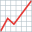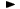We could not find any results for:

### ToplistsIt looks like you aren't logged in.

Subscribe Login/RegisterRegister for streaming realtime charts, analysis tools, and prices.
ADVFN HomeHelpFinancialsDeeper AnalysisOPERATING RATIOS - Profitability RatiosDividend Payout Ratio

# Dividend Payout Ratio

Dividend Payout ratio, measures the proportion of earnings that is paid out as dividends.

It is calculated as the annual total dividend amount per share divided by the earnings per share-basic (EPS-basic). Thus, the formula is the following:

= (total annual dividend / EPS-basic) * 100

Note: If the company had losses during the period under review, Dividend Payout ratio is not defined.